Select Page

# Introduction:

All of us are aware of the concept of the median in Statistics, the middle value or the mean of the two middle values, of an array. We have learned that the median divides a set of data into two equal parts. In the same way, there are also certain other values which divide a set of data into four, ten or hundred equal parts. Such values are referred as quartiles, deciles, and percentiles respectively.

Collectively, the quartiles, deciles and percentiles and other values obtained by equal sub-division of the data are called Quartiles.

## Quartiles:

The values which divide an array (a set of data arranged in ascending or descending order) into four equal parts are called Quartiles. The first, second and third quartiles are denoted by Q1, Q2,Q3 respectively. The first and third quartiles are also called the lower and upper quartiles respectively. The second quartile represents the median, the middle value.

Are you interested in creating an Academic Blog just like econtutorials.com ? Check my detailed step-by-step tutorials of creating your own blog in 2018.

### Quartiles for Ungrouped Data:

Quartiles for ungrouped data are calculated by the following formulae.

## For Example:

Following is the data of marks obtained by 20 students in a test of statistics;

 53 74 82 42 39 20 81 68 58 28 67 54 93 70 30 55 36 37 29 61

In order to apply formulae, we need to arrange the above data into ascending order i.e. in the form of an array.

 20 28 29 30 36 37 39 42 53 54 55 58 61 67 68 70 74 81 82 93

Here, n = 20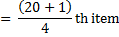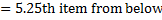The value of the 5th item is 36 and that of the 6th item is 37. Thus, the first quartile is a value 0.25th of the way between 36 and 37, which are 36.25. Therefore,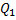= 36.25. Similarly,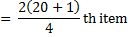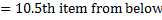The value of the 10th item is 54 and that of the 11th item is 55. Thus the second quartile is the 0.5th of the value 54 and 55. Since the difference between 54 and 55 is of 1, therefore 54 + 1(0.5) = 54.5. Hence,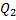= 54.5. Likewise,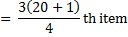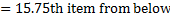The value of the 15th item is 68 and that of the 16th item is 70. Thus the third quartile is a value 0.75th of the way between 68 and 70. As the difference between 68 and 70 is 2, so the third quartile will be 68 + 2(0.75) = 69.5. Therefore,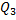= 69.5.

## Quartiles for Grouped Data:

The quartiles may be determined from grouped data in the same way as the median except that in place of n/2 we will use n/4. For calculating quartiles from grouped data we will form cumulative frequency column. Quartiles for grouped data will be calculated from the following formulae;

Where,

l = lower class boundary of the class containing the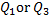, i.e. the class corresponding to the cumulative frequency in which n/4 or 3n/4 lies

h = class interval size of the class containing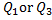.

f = frequency of the class containing.

n = number of values, or the total frequency.

C.F = cumulative frequency of the class preceding the class containing.

### For Example:

We will calculate the quartiles from the frequency distribution for the weight of 120 students as given in the following Table 18;

Table 18
 Weight (lb) Frequency (f) Class Boundaries Cumulative Frequency 110 – 119 1 109.5 – 119.5 0 120 – 129 4 119.5 – 129.5 5 130 – 139 17 129.5 – 139.5 22 140 – 149 28 139.5 – 149.5 50 150 – 159 25 149.5 – 159.5 75 160 – 169 18 159.5 – 169.5 93 170 – 179 13 169.5 – 179.5 106 180 – 189 6 179.5 – 189.5 112 190 – 199 5 189.5 – 199.5 117 200 – 209 2 195.5 – 209.5 119 210 – 219 1 209.5 – 219.5 120 ∑f = n = 120

i. The first quartileis the value of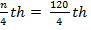or the 30th item from the lower end. From Table 18 we see that cumulative frequency of the third class is 22 and that of the fourth class is 50. Thuslies in the fourth class i.e. 140 – 149.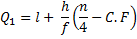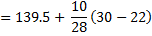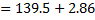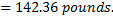ii. The thirds quartileis the value of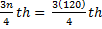or 90th item from the lower end. The cumulative frequency of the fifth class is 75 and that of the sixth class is 93. Thus,lies in the sixth class i.e. 160 – 169.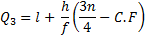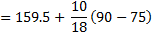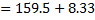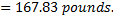### Conclusion

From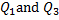we conclude that 25% of the students weigh 142.36 pounds or less and 75% of the students weigh 167.83 pounds or less.## Deciles:

The values which divide an array into ten equal parts are called deciles. The first, second,…… ninth deciles by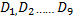respectively. The fifth decile (corresponds to median. The second, fourth, sixth and eighth deciles which collectively divide the data into five equal parts are called quintiles.

### Deciles for Ungrouped Data:

Deciles for ungrouped data will be calculated from the following formulae;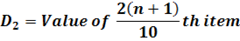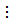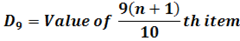#### For Example:

We will calculate second, third and seventh deciles from the following array of data.
 20 28 29 30 36 37 39 42 53 54 55 58 61 67 68 70 74 81 82 93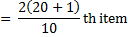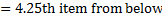The value of the 4th item is 30 and that of the 5th item is 36. Thus the second decile is a value 0.2th of the way between 30 and 36. The fifth decile will be 30 + 6(0.2) = 31.2. Therefore,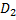= 31.2.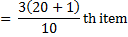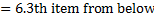The value of the 6th item is 37 and that of the 7th item is 39. Thus the third decile is 0.3th of the way between 37 and 39. The third decile will be 37 + 2(0.3) = 37.6. Hence,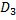= 37.6.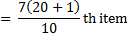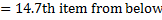The value of the 14th item is 67 and that of the 15th item is 68. Thus the 7th decile is 0.7th of the way between 67 and 68, which will be as 37 + 0.7 = 67.7. Therefore,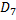= 67.7.

## Decile for Grouped Data

Decile for grouped data can be calculated from the following formulae;

Where,

l = lower class boundary of the class containing the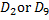, i.e. the class corresponding to the cumulative frequency in which 2n/10 or 9n/10 lies

h = class interval size of the class containing.

f = frequency of the class containing.

n = number of values, or the total frequency.

C.F = cumulative frequency of the class preceding the class containing.

### For Example:

We will calculate fourth, seventh and ninth deciles from the frequency distribution of weights of 120 students, as provided in Table 18.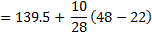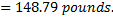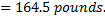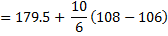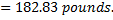#### Conclusion:

From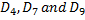we conclude that 40% students weigh 148.79 pounds or less, 70% students weigh 164.5 pounds or less and 90% students weigh 182.83 pounds or less.## Percentiles:

The values which divide an array into one hundred equal parts are called percentiles. The first, second,……. Ninety-ninth percentile are denoted by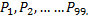The 50th percentile (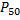) corresponds to the median. The 25th percentile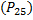corresponds to the first quartile and the 75th percentile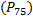corresponds to the third quartile.

## Percentiles for Ungrouped Data:

Percentile from ungrouped data could be calculated from the following formulae;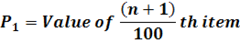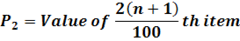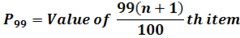For Example:

We will calculate fifteenth, thirty-seventh and sixty-fourth percentile from the following array;

 20 28 29 30 36 37 39 42 53 54 55 58 61 67 68 70 74 81 82 93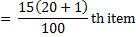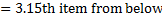The value of the 3rd item is 29 and that of the 4th item is 30. Thus the 15th percentile is 0.15th item the way between 29 and 30, which will be calculated as 29 + 0.15 = 29.15. Hence,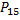= 29.15.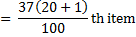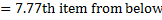The value of 7th item is 39 and that of the 8th item is 42. Thus the 37th percentile is 0.77th of the between 39 and 42, which will be calculate as 39 + 3(0.77) = 41.31. Hence,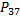= 41.31.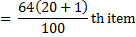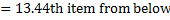The value of the 13th item is 61 and that of the 14th item is 67. Thus, the 64th percentile is 0.44th of the way between 61 and 67. Since the difference between 61 and 67 is 6 so 64th percentile will be calculated as 61 + 6(0.44) = 63.64. Hence,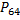= 63.64.

## Percentiles for Grouped Data:

Percentiles can also be calculated for grouped data which is done with the help of following formulae;

Where,

l = lower class boundary of the class containing the, i.e. the class corresponding to the cumulative frequency in which 35n/100 or 99n/100 lies

h = class interval size of the class containing.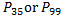.

f = frequency of the class containing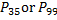.

n = number of values, or the total frequency.

C.F = cumulative frequency of the class preceding the class containing.

### For Example:

We will calculate thirty-seventh, forty-fifth and ninetieth percentile from the frequency distribution of weights of 120 students, by using the Table 18.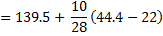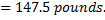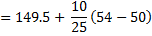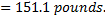### Conclusion

From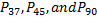we have concluded or interpreted that 37% student weigh 147.5 pounds or less. Similarly, 45% students weigh 151.1 pounds or less and 90% students weigh 182.83 pounds or less.

Are you interested in creating an Academic Blog just like econtutorials.com ? Check my detailed step-by-step tutorials of creating your own blog in 2018.

Shares